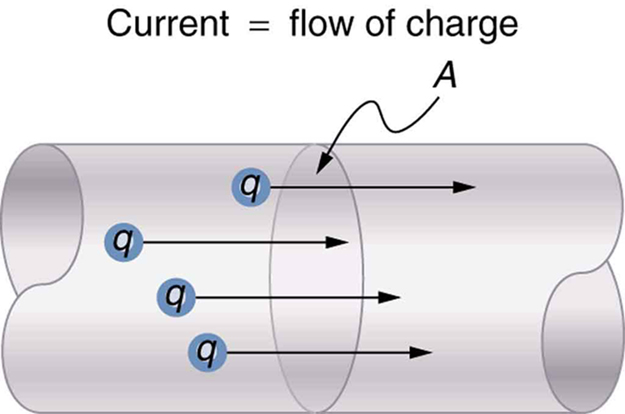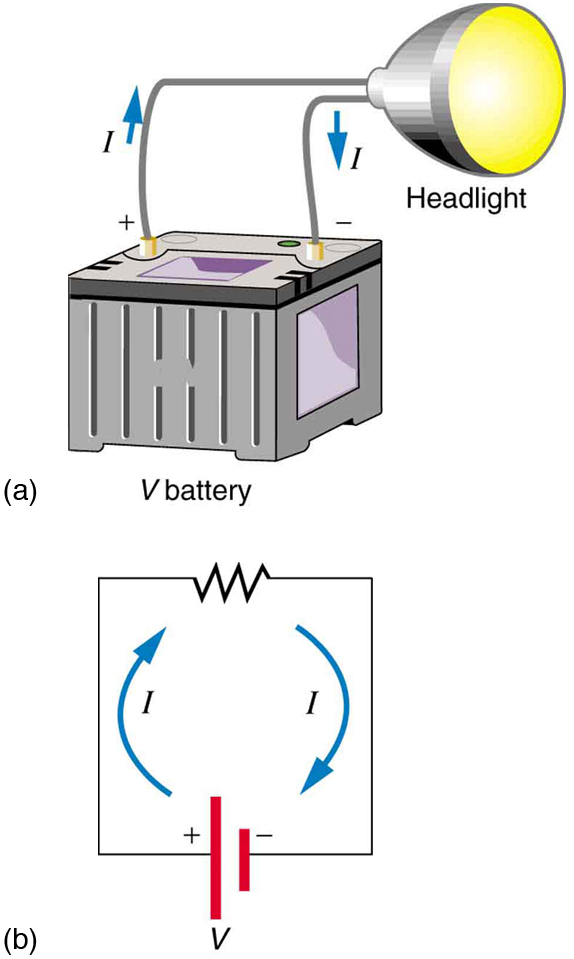## Overview of Electric Current

Electric current is the flow of electric charge and resistance is the opposition to that flow.

### Learning Objectives

Explain difference between conductor and resistor

### Key Takeaways

#### Key Points

• Electric current is the movement of electric charge through a conductive medium.
• We also use the term “current” as a quantity to describe the rate at which charge flows through a medium. The SI unit for current is the ampere (A), which is equal to a coulomb per second (C/s).
• Conductance is a quantity describing how easily charge can flow through a material, while resistance is the inverse, a measure of how strongly a material opposes electric flow.
• An object that allows charge to flow easily is called a conductor, while an object that resists the flow of charge is called a resistor.

#### Key Terms

• conductive medium: A material that can transmit electricity.
• electrical resistance: The opposition offered by an electrical conductor to the flow of a current through itself, resulting in a conversion of electrical energy into heat and radiation. The SI derived unit of resistance is the ohm. Symbol: R.
• electric charge: A quantum number that determines the electromagnetic interactions of some subatomic particles; by convention, the electron has an electric charge of -1 and the proton +1, and quarks have fractional charge.

### Introduction to Electric Current and Resistance

From ceiling lights to circuit chips, from power steering to Internet browsing, electricity provides the basis for our technology and civilization. The firing of neurons in your brain is also an example of electric current – that is, the movement of electric charge through a conductive medium. In electric circuits, this charge is often carried by moving electrons in a wire. It can also be carried by ions in an electrolyte, or by both ions and electrons such as in a plasma.

### Electric Current

When we speak of electric current, often we are referring to a specific quantity – the rate at which charge flows. A large current, such as that used to start a truck engine, moves a large amount of charge in a small time, whereas a small current, such as that used to operate a hand-held calculator, moves a small amount of charge over a long period of time. In equation form, electric current I is defined to be

$\text{I}=\frac{\Delta \text{Q}}{\Delta \text{T}}$

where Q is the amount of charge passing through a given area in time t. The SI unit for current is the ampere (A), named for the French physicist André-Marie Ampère (1775–1836). Since I=ΔQ/Δt, we see that an ampere is one coulomb per second:

$1 \ \text{A} = 1 \ \text{C}/\text{s}$

The flow of electricity requires a medium in which charge can flow. We call an object or medium that allows charge to flow a conductor, while the empirical measure of a material’s ability to conduct charge is called the electrical conductance. The SI unit for conductance is the siemens (S).Electric Current: The rate of flow of charge is current. An ampere is the flow of one coulomb through an area in one second.

### Resistance

The opposite of conductance is resistance – a quantity that describes how strongly a material opposes the flow of electric current. An object or medium that has high electrical resistance is called a resistor. We will see that the resistance of an object depends on its shape and the material of which it is composed. The SI unit for resistance is the ohm (symbol: ).

### Electric Circuits

A useful and practical way to learn about electric current and resistance is to study circuits. The figure above shows a simple circuit and the standard schematic representation of a battery, conducting path, and load (a resistor). Schematics are very useful in visualizing the main features of a circuit. A single schematic can represent a wide variety of situations. The schematic in (b), for example, can represent anything from a truck battery connected to a headlight lighting the street in front of the truck to a small battery connected to a penlight lighting a keyhole in a door. Such schematics are useful because the analysis is the same for a wide variety of situations. We need to understand a few schematics to apply the concepts and analysis to many more situations.Simple Electric Circuit: (a) A simple electric circuit. A closed path for current to flow through is supplied by conducting wires connecting a load to the terminals of a battery. (b) In this schematic, the battery is represented by the two parallel red lines, conducting wires are shown as straight lines, and the zigzag represents the load. The schematic represents a wide variety of similar circuits.

Note that the direction of current flow in the figure is from positive to negative. The direction of conventional current is the direction that positive charge would flow. Depending on the situation, positive charges, negative charges, or both may move. In metal wires, for example, current is carried by electrons—that is, negative charges move. In ionic solutions, such as salt water, both positive and negative charges move.

It is important to realize that there is an electric field in conductors responsible for producing the current. Unlike static electricity, where a conductor in equilibrium cannot have an electric field in it, conductors carrying a current have an electric field and are not in static equilibrium. An electric field is needed to supply energy to move the charges.

Armed with these basics, we’ll begin to tackle the harder details of this topic in the next section.